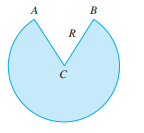Chapter 3.7, Problem 41E

Chapter
Section
Textbook Problem

# A cone-shaped drinking cup is made from a circular piece of paper of radius R by cutting out a sector and joining the edges CA and CB. Find the maximum capacity of such a cup.To determine

To find:

The maximum capacity of a cup.

Explanation

1) Concept:

i) A critical number of a function f   is a number c in the domain of f  Such that either  f'c=0 or f'c does not exist.

ii) First derivative test for absolute extreme values- suppose that c is a critical number of a continuous function f defined on an interval.

If f'x>0 for all x<c and f'x<0 for all >c, then fc is the absolute maximum value of f.

If f'x<0 for all x<c and f'x>0 for all >c, then fc is the absolute Minimum value of f.

2) Formula:

Volume of a cone:V=π3r2h

3) Given:

A cone shaped drinking cup is made up of a circular piece of paper of radius R by cutting out a sector and joining the edge CA and CB.

4) Calculation:

As a cone shaped drinking cup is made up of circular piece of paper of radius R by cutting out a sector and joining the edge CA and CB, therefore, it looks like,

By using Pythagoras theorem, R2= r2+h2

Therefore, r2=R2-h2

As volume of cone =π3r2h= π3(R2-h2)h

V=π3(R2h- h3)

By definition of critical points,

Therefore, differentiating V with respect to h,

V'h=π3(R2-3h2)

Volume is maximum or minimum when V'h=0.

Therefore,π3R2-3h2=0

R2-3h2=0 as π30

R2=3h2

h2=13R2

By taking square roots on both sides,

h=13R

for 0<h<13

### Still sussing out bartleby?

Check out a sample textbook solution.

See a sample solution

#### The Solution to Your Study Problems

Bartleby provides explanations to thousands of textbook problems written by our experts, many with advanced degrees!

Get Started